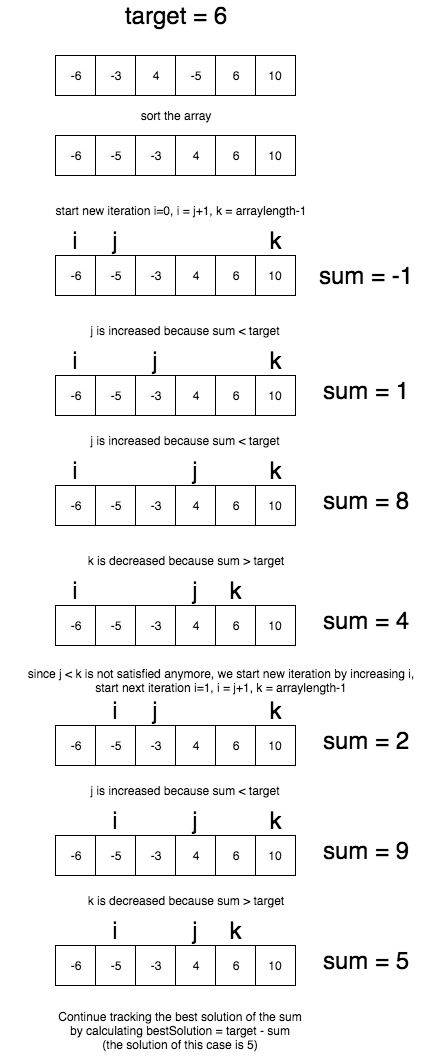Given an array nums of n integers and an integer target, find three integers in nums such that the sum is closest to target. Return the sum of the three integers. You may assume that each input would have exactly one solution. from leetcode

Example:

``````Given array nums = [-1, 2, 1, -4], and target = 1.
The sum that is closest to the target is 2. (-1 + 2 + 1 = 2).
``````

# Approach

1. Sort the input array
2. Iterate i over range 0 to nums.length-2
3. While other j and k scanning remaining part of the array by approaching each other
4. The condition for j and k scanners to find the `sum = nums[i] + nums[j] + nums[k]` closest to given target
5. If the sum is less than target then we should increase j
6. If the sum is greater than target then we should decrease k# Java Solution

``````class Solution {
public int threeSumClosest(int[] nums, int target) {
if (nums == null || nums.length < 3) {
return Integer.MAX_VALUE;
}
Arrays.sort(nums);
int bestSolution = nums + nums + nums;
for (int i = 0; i < nums.length - 2 ; i++) {
int j = i+1;
int k = nums.length - 1;

while (j < k) {
int curSum = nums[i] + nums[j] + nums[k];
if (Math.abs(target - bestSolution) > Math.abs(target-curSum)) {
bestSolution = curSum;
}
if (curSum < target) {
j++;
} else if (curSum > target) {
k--;
} else {
return target;
}
}
}
return bestSolution;
}
}
``````